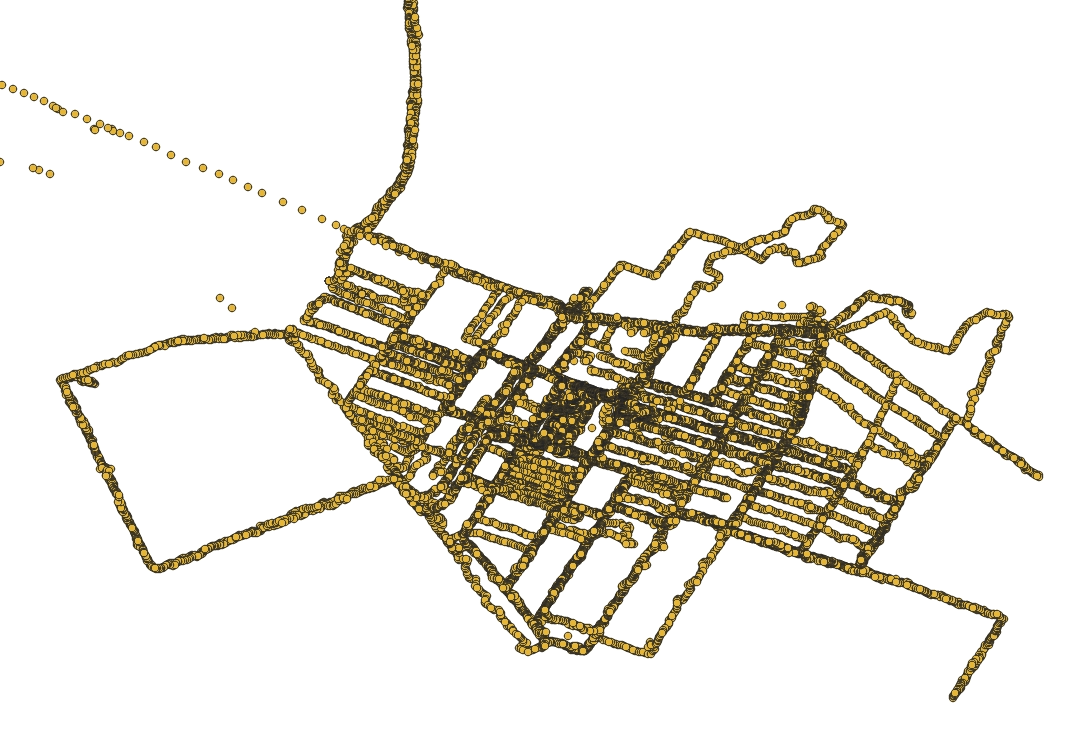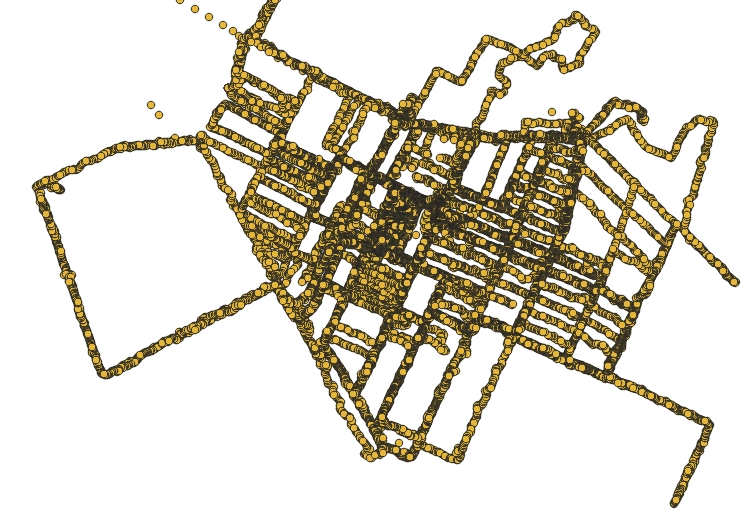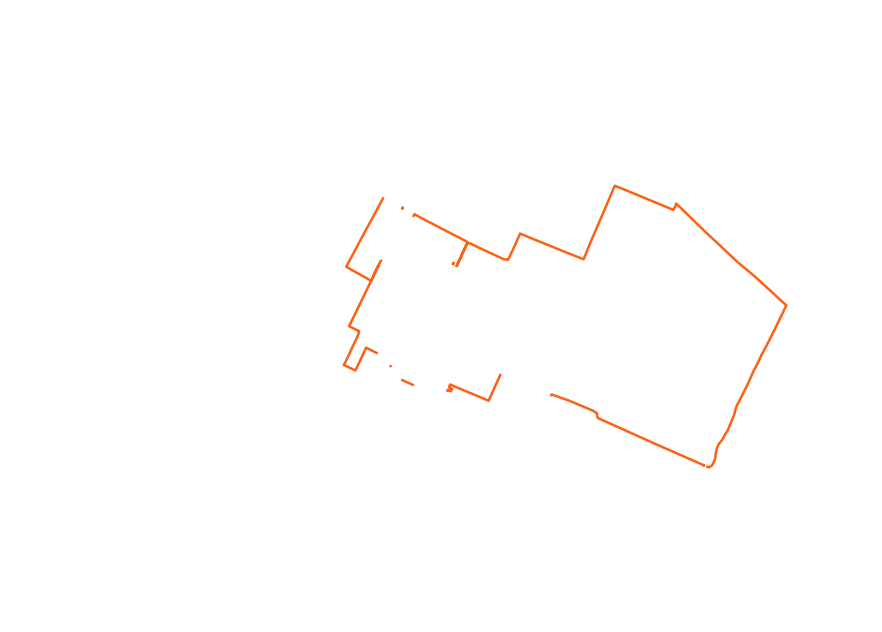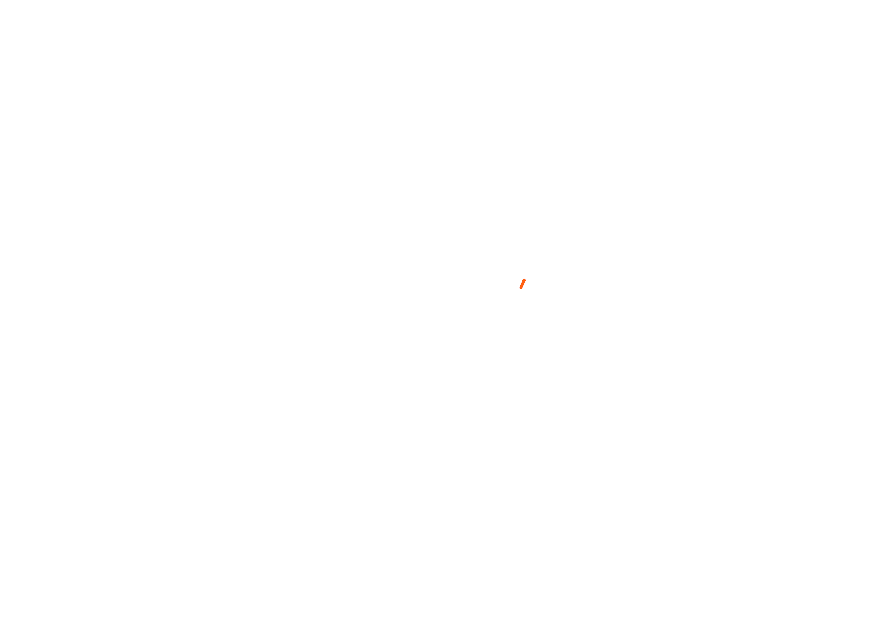# Mapping my walks with OSRM and Rust

Last week, we looked at the data from my smartwatch app and how to snap it to the OpenStreetMap roads. If you haven't already, consider reading that post before moving on. This time, we'll be writing some code in the Rust programming language. Keep in mind that this isn't exactly production-grade code, but rather something you would normally write in Python in one afternoon. I'll link to the crates I'm using for the benefit of readers less familiar with the Rust ecosystem.

## Importing the data

If you recall from last time, our data points include a timestamp, the latitude and longitude, and an accuracy radius. We can define our point type as follows:

``````pub struct Point {
pub time: OffsetDateTime,
pub geom: geo_types::Point<f64>,
}
``````

The `OffsetDateTime` and `Point<T>` types are from the `time` and `geo-types` crates, respectively. We'll also need `zip` and `csv` in order to parse the exported file.

Let's see what reading the file looks like:

``````pub fn read_archive(path: &Path) -> Result<Vec<Point>, Error> {
let file = File::open(path)?;
let mut archive = ZipArchive::new(file)?;
let mut latitudes = Vec::new();
let mut record = StringRecord::new();
{
latitudes.push(get_array_first_value(&record)?);
}
}
let n = latitudes.len();
let mut times = Vec::with_capacity(n);
let mut longitudes = Vec::with_capacity(n);
{
let time = OffsetDateTime::parse(&record, &well_known::Rfc3339)?;
times.push(time);
longitudes.push(get_array_first_value(&record)?);
}
}
assert_eq!(longitudes.len(), n);
{
}
}
let points = izip!(times, latitudes, longitudes, radiuses)
let geom = geo_types::Point::new(lon, lat);
Point { time, geom, radius }
})
.collect();
Ok(points)
}

fn get_array_first_value<T>(s: &str) -> Result<T, T::Err>
where
T: FromStr,
T::Err: std::fmt::Debug,
{
let p = s.find(|c| c == ']' || c == ',').expect("expected array");
s[1..p].parse()
}
``````

The code is somewhat unwieldly because it needs to read three different CSVs from the archive. The ZIP reader needs to seek into the archive, so we can't read all of them at once. If you're familiar with Rust, that constraint is expressed in the type system by `Reader` having a mutable reference to `ZipArchive`. Instead, we open the files one at a time and scan through each.

We need to remember to read the timestamps and there is one extra complication. The values are written as JSON-like arrays (e.g. `[44.XXX,657.XXX]`), but only the first one makes sense. Instead of reaching for a full-blown JSON parser, we simply look for a bracket or comma and parse the number we find there.

The `izip` macro comes from `itertools` and lets us iterate over the multiple collections at once.

Note that the implementation keeps all the points in memory (twice, even). Normally I would dump the points to disk and read them back, but it would make the code harder to follow in a blog post. In any case, this doesn't dominate our memory usage.

## Calling into OSRM

As mentioned last time, OSRM has an HTTP server, so we'll use `reqwest` to call into it and `serde` to deserialize the JSON responses.

First of all, we define some structs that roughly match the OSRM response:

``````#[derive(Deserialize, Debug)]
pub struct MatchResponse {
pub matchings: Vec<Matching>,
}

#[derive(Deserialize, Debug)]
pub struct Matching {
pub geometry: String,
}
``````

Then our client:

``````pub struct OsrmClient {
client: Client,
base_url: String,
}

impl OsrmClient {
pub fn new(base_url: String) -> Self {
let client = Client::new();
Self { client, base_url }
}

pub fn match_map(
&self,
profile: &str,
points: &[Point],
) -> Result<MultiLineString<f64>, Error> {
let mut q = format!("{}match/v1/{profile}/polyline6(", self.base_url);
let mut timestamps = String::new();
let coordinates = LineString::from_iter(points.iter().map(|p| p.geom));
for point in points {
write!(timestamps, "{};", point.time.unix_timestamp())?;
}
timestamps.pop();
let coordinates =
polyline::encode_coordinates(coordinates, 6).map_err(|e| anyhow!(e))?;
let pe = percent_encoding::percent_encode(
coordinates.as_bytes(),
percent_encoding::NON_ALPHANUMERIC,
);
write!(
q,
"{})?geometries=polyline6&tidy=true&steps=false&timestamps=",
pe
)?;
q.push_str(&timestamps);
let res = self.client.get(&q).send()?.json::<MatchResponse>()?;
let mls = MultiLineString(
res.matchings
.into_iter()
.map(|m| polyline::decode_polyline(&m.geometry, 6))
.collect::<Result<Vec<_>, _>>()
.map_err(|e| anyhow!(e))?,
);
Ok(mls)
}
}
``````

Again, this is quick and dirty code. We use the `polyline` encoding of the coordinates and the corresponding crate for it. Because `polyline` uses an encoding that contains non-alphanumeric characters, we need to encode them using `percent-encoding`. This crate doesn't have a standard error type (it uses `String`s), so we adapt them using `anyhow`, which is also appears in the rest of the code.

## Saving a FlatGeobuf

This isn't really required for our purposes, but I want to keep a copy of the points in a format better suited for using later. I'd normally use GeoPackage, but I want to give FlatGeobuf a try. This is a newer format, with a simpler structure and which should be simpler to write, since a pure-Rust implementation is available. Fortunately, GDAL also has a reader, so it should be compatible with every application I care about at the moment.

`flatgeobuf` has a slightly strange API, but it's not too bad and I've seen worse. It integrates closely with `geozero`, which is a visitor-based API for zero-copy processing of geospatial data.

``````fn export_fgb(name: &str, file: &Path, points: &[Point]) -> anyhow::Result<()> {
let mut fgb = FgbWriter::create(name, GeometryType::Point, |_, _| {})?;
fgb.set_crs(4326, |_fbb, _crs| {});
col.nullable = false;
});
col.nullable = false;
});
for point in points {
let time = point.time.format(&well_known::Rfc3339)?;
feat.property(0, "time", &ColumnValue::DateTime(&time))
.unwrap();
.unwrap();
})?;
}
let out_file = File::create(file)?;
fgb.write(&mut BufWriter::new(out_file))?;
Ok(())
}
``````

We create a writer, configure the CRS and define the two columns. Afterwards, we can iterate over our points, create features from them, and set the column values.

## Reprojecting the data

I want to display the resulting tracks on a map, but the (latitude, longitude) coordinates aren't ideal, since they introduce quite a bit of distorsion:Instead, we will reproject our points to the Web Mercator (or, more formally, EPSG:3857) projection. While it's not really accurate, it's good enough at this latitude for my purposes. Web Mercator has been popularized by Google Maps, and the look of it should be familiar to anyone who's ever seen an interactive map on the Web.For this, I'd normally use `GDAL` or `PROJ`, but GDAL's approach to threading isn't the most fortunate. We could probably use the `proj` bindings, but even those bring quite a bit of complexity.

The conversion to Web Mercator should be pretty easy. You can see a pair of formulas in the link above, but they use different bounds, and it's not obvious how to do it correctly. Stack Overflow produces a fair bit of confusion and two answers, but neither of them agrees with `PROJ`. After a bit of hit-and miss, I realized that the three implementations are using different values for the Earth radius. I believe the correct value to use in this case is `6_378_137 m`. My final implementation gives the same result (up to the precision limit) as `PROJ` for a couple of points I've tried, so I hope it's correct.

``````pub fn epsg_4326_to_3857(x: f64, y: f64) -> (f64, f64) {
const MAX_LATITUDE: f64 = 85.06;

let y = if y > MAX_LATITUDE {
std::f64::consts::PI
} else if y < -MAX_LATITUDE {
-std::f64::consts::PI
} else {
let y = y.to_radians() / 2.0 + std::f64::consts::FRAC_PI_4;
y.tan().ln()
};
}
``````

There is also a helper that reprojects an entire track:

``````fn reproject_route(mls: &mut MultiLineString<f64>) {
mls.iter_mut().for_each(|ls| {
ls.0.iter_mut().for_each(|p| {
let (x, y) = geo::epsg_4326_to_3857(p.x, p.y);
p.x = x;
p.y = y;
});
});
}
``````

## Rasterization

Next, I want to display the tracks returned by OSRM as images. Converting from vector data to an image is called rasterization. I had a couple of options here (it's less flexible, but GDAL can do it), but I decided to a recently-published crate called `rasterize`.

First of all, `rasterize` represents paths as a list of segments, not points, so we need a conversion function for that:

``````fn mls_to_path(mls: MultiLineString<f64>) -> rasterize::Path {
let subpaths = mls
.into_iter()
.filter_map(|ls| {
SubPath::new(
ls.into_iter()
.map(|p| p.x_y())
.tuple_windows()
.map(|(p1, p2)| Segment::Line(Line::new(p1, p2)))
.collect(),
false,
)
})
.collect();
rasterize::Path::new(subpaths)
}
``````

We also need to pick a couple of things:

• the view bounding box in map coordinates
• the stroke style and color
• a scale factor to apply, so that our output image size doesn't depend on the EPSG:3857 coordinates
``````struct RasterizeOptions {
rasterizer: ActiveEdgeRasterizer,
bbox: BBox,
transform: Transform,
stroke_color: Arc<LinColor>,
stroke_style: StrokeStyle,
}
``````

Then we can write a bit of code to rasterize a track and save the result to a file:

``````fn rasterize_route(
options: &RasterizeOptions,
output: File,
mut mls: MultiLineString<f64>,
) -> anyhow::Result<()> {
reproject_mls(&mut mls);
let scene = Scene::stroke(
Arc::new(mls_to_path(mls)),
Arc::clone(&options.stroke_color) as Arc<dyn Paint>,
options.stroke_style,
);
let layer = scene.render(
&options.rasterizer,
options.transform,
Some(options.bbox),
None,
);
layer.write_png(output)?;
Ok(())
}
``````

The result looks like this (if it looks weird for you, it's because it has a transparent background):## Accumulating the images

At the end, I want to overlay all the tracks in the same image. We load the files from the previous step using `png`, then accumulate them into a white layer:

``````fn accumulate_images(bbox: BBox, images: &[String]) -> anyhow::Result<()> {
let mut layer = Layer::new(bbox, Some(LinColor::new(1.0, 1.0, 1.0, 1.0)));
let mut buf = vec![0; layer.width() * layer.height() * 4];
for img in images {
{
let decoder = Decoder::new(File::open(&img)?);
layer
.iter_mut()
.zip((&buf[..info.buffer_size()]).chunks(4))
.for_each(|(pa, p)| {
let p = &p[..4];
*pa = pa.blend_over(&ColorU8::new(p, p, p, p).into());
});
}
let file = File::create(img)?;
layer.write_png(file)?;
}
Ok(())
}
``````

The bleding operator (`blend_over`, from `rasterize`) keeps the existing pixels and only overwrites the transparent ones.

## Putting it together

Skipping over argument parsing and other initializations, we can finally read the archive and save the FlatGeobuf file:

``````let mut points = import::read_archive(archive)?;
export_fgb("location", &archive.with_extension("fgb"), &points)?;
points.sort_unstable_by_key(|p| p.time);
``````

We then filter out the bad points and group them by date:

``````let points = points
.into_iter()
.group_by(|p| p.time.date())
.into_iter()
.map(|(d, p)| (d, p.collect::<Vec<_>>()))
.collect::<Vec<_>>();
``````

Run them through OSRM:

``````let osrm_client = osrm::OsrmClient::new("http://127.0.0.1:5000/".to_string());
let routes = points
.into_par_iter()
.filter_map(|(date, points)| {
osrm_client
.match_map("foot", &points)
.ok()
.map(|m| (date, m))
})
.collect::<Vec<_>>();
``````

I'm using `rayon` here in order to do multiple requests to OSRM at once. It would be very inconsiderate to do this against another server, but I'm running my own instance in Docker.

Rasterize the routes for every date into a corresponding image:

``````let mut images = routes
.into_par_iter()
.map(|(date, mls)| {
let raster_name = format!("{date}.png");
let file = File::create(&raster_name)?;
rasterize_route(&rasterize_options, file, mls)?;
Ok(raster_name)
})
.collect::<Result<Vec<_>, Error>>()?;
``````

Again, this uses `rayon` for parallelization. Since I mentioned the memory usage before, there is one subtle issue here. Every running thread will rasterize some tracks into a layer, so the memory usage will depend on the available concurrency. If some computation takes, say, 1 GB RAM, that's not too bad until you start 32 of them at once. So remember to pair CPUs with many cores with an appropriate amount of RAM, even if it sits mostly unused.

Then we finally run the accumulation step:

``````images.sort_unstable();
accumulate_images(bbox, &images)?;
``````

And just one thing left to do:

``````\$ ffmpeg -framerate 15 -pattern_type glob -i '*.png' -c:v libwebp_anim -lossless 1 -quality 100 gps_tracks.webp
``````

You can see the result below:## Performance

I've only done rough measurements, but the whole process (except running `ffmpeg` at the end) takes 4.5 seconds on my system. Replacing `into_par_iter` with `into_iter` brings that up to 10.5 seconds.

Out of the 4.5 s, calling into OSRM takes 1.3 s (6.5 s serially). The rest of the time is spent in rasterization (which is quite fast), and PNG compression and decompression.

## Closing words

If you've followed through, thank you for reading. You can find the code on GitHub. Props also to the GeoRust community, which owns all the geospatial-related crates I've used here.

Map data from OpenStreetMap